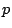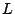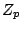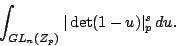# $p$--adic random matrix theory

Can-adic random matrix theory explain the arithmetic factors that appear in the asymptotic formulas for the mean values of-functions?

It would be interesting to evaluate moments of the-adic absolute value of the characteristic polynomial of matrices averaged over the classical groups (over). In other words, evaluate(More information is sought. It was suggested that these calculations may have already been done by Anderson(?) or they may be in Macdonald's book?)

Back to the main index for L-functions and Random Matrix Theory.